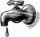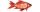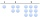# Fraction calculator

The calculator performs basic and advanced operations with fractions, expressions with fractions combined with integers, decimals, and mixed numbers. It also shows detailed step-by-step information about the fraction calculation procedure. Solve problems with two, three, or more fractions and numbers in one expression.

## Result:

### 2/3 * 1/2 = 1/3 ≅ 0.3333333

Spelled result in words is one third.

### How do you solve fractions step by step?

1. Multiple: 2/3 * 1/2 = 2 · 1/3 · 2 = 2/6 = 1 · 2/3 · 2 = 1/3
Multiply both numerators and denominators. Result fraction keep to lowest possible denominator GCD(2, 6) = 2. In the following intermediate step, cancel by a common factor of 2 gives 1/3.
In other words - two thirds multiplied by one half = one third.

#### Rules for expressions with fractions:

Fractions - simply use a forward slash between the numerator and denominator, i.e., for five-hundredths, enter 5/100. If you are using mixed numbers, be sure to leave a single space between the whole and fraction part.
The slash separates the numerator (number above a fraction line) and denominator (number below).

Mixed numerals (mixed fractions or mixed numbers) write as integer separated by one space and fraction i.e., 1 2/3 (having the same sign). An example of a negative mixed fraction: -5 1/2.
Because slash is both signs for fraction line and division, we recommended use colon (:) as the operator of division fractions i.e., 1/2 : 3.

Decimals (decimal numbers) enter with a decimal point . and they are automatically converted to fractions - i.e. 1.45.

The colon : and slash / is the symbol of division. Can be used to divide mixed numbers 1 2/3 : 4 3/8 or can be used for write complex fractions i.e. 1/2 : 1/3.
An asterisk * or × is the symbol for multiplication.
Plus + is addition, minus sign - is subtraction and ()[] is mathematical parentheses.
The exponentiation/power symbol is ^ - for example: (7/8-4/5)^2 = (7/8-4/5)2

#### Examples:

subtracting fractions: 2/3 - 1/2
multiplying fractions: 7/8 * 3/9
dividing Fractions: 1/2 : 3/4
exponentiation of fraction: 3/5^3
fractional exponents: 16 ^ 1/2
adding fractions and mixed numbers: 8/5 + 6 2/7
dividing integer and fraction: 5 ÷ 1/2
complex fractions: 5/8 : 2 2/3
decimal to fraction: 0.625
Fraction to Decimal: 1/4
Fraction to Percent: 1/8 %
comparing fractions: 1/4 2/3
multiplying a fraction by a whole number: 6 * 3/4
square root of a fraction: sqrt(1/16)
reducing or simplifying the fraction (simplification) - dividing the numerator and denominator of a fraction by the same non-zero number - equivalent fraction: 4/22
expression with brackets: 1/3 * (1/2 - 3 3/8)
compound fraction: 3/4 of 5/7
fractions multiple: 2/3 of 3/5
divide to find the quotient: 3/5 ÷ 2/3

The calculator follows well-known rules for order of operations. The most common mnemonics for remembering this order of operations are:
PEMDAS - Parentheses, Exponents, Multiplication, Division, Addition, Subtraction.
BEDMAS - Brackets, Exponents, Division, Multiplication, Addition, Subtraction
BODMAS - Brackets, Of or Order, Division, Multiplication, Addition, Subtraction.
GEMDAS - Grouping Symbols - brackets (){}, Exponents, Multiplication, Division, Addition, Subtraction.
Be careful, always do multiplication and division before addition and subtraction. Some operators (+ and -) and (* and /) has the same priority and then must evaluate from left to right.

## Fractions in word problems:

• Tallest and shortestJenn is the tallest on the team. He is 1 1/2 times as tall as the shortest girl whom is 4 1/4 feet tall. How tall is Jebb?
• Ms. SheppardMs. Sheppard cuts ½ of a piece of construction paper. She uses ⅙ of the piece to make a flower. What fraction of the sheet of paper does she use to make the flower?
• One third 2One third of all students in class live in a house. If here are 42 students in a class, how many of them live in house?
• The tapFor one day flows 148 l of water out of the tap. How much water will flow out for 3/4 day?
• Yellow tangsIn an aquarium 2/5 of the fish are surgeonfish.  Of these 3/4 are yellow tangs.  What fraction of all fish in the aquarium are yellow tangs?
• Unknown number4/5 of a number is 276. what is 2/3 of the same number?
• Quotient 3If the quotient of 8/13 and 2 is subtracted from the product of 1 3/4 and 8/21, what is the difference?
• CerealsAri and Joey share a 30-ounce box of cereal. By the end of the week, Ari has eaten 3/10 of the box, and Joey has eaten 3/5 of the box of cereal. How many ounces are left in the box?
• There 14There are 250 people in a museum. 2/5 of the 250 people are girls 3/10 of the 250 people are boys The rest of the 250 people are adults Work out the number of adults in the museum.
• KennethKenneth is painting his kitchen and bathroom. He bought 5 gallons of paint to paint the two rooms. He uses 1/4 of that amount to paint the bathroom and the rest to paint the kitchen. How many gallons of paint did Kenneth use to paint the kitchen?
• Evaluate 17Evaluate 2x+6y when x=- 4/5 and y=1/3. Write your answer as a fraction or mixed number in simplest form.
• Length 7Length equals 7/6 inch in width equals 7/9 inches the area is?
• Gear wheelsTwo gear wheels, which fit together, have the number of teeth z1=58 and z2=149. Calculate the speed of the first wheel, if the second wheel rotates 1232 revolutions per minute.